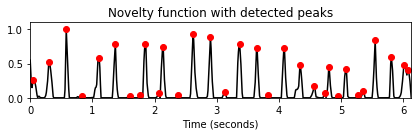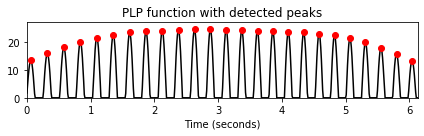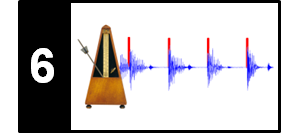# Predominant Local Pulse (PLP)

Following Section 6.3.1 of [Müller, FMP, Springer 2015], we introduce in this notebook an algorithm to compute predominant local pulse (PLP). This concept was first described by Grosche and Müller. A MATLAB implementation can be found in the Tempogram Toolbox. An application and analysis of the PLP technique within a challenging music scenario can found in the article on "What makes beat tracking difficult?"

## Main Idea¶

The task of beat and pulse tracking can be seen as an extension of tempo estimation in the sense that, additionally to the rate, it also considers the phase of the pulses. The idea of the Fourier Tempogram was to locally compare a given novelty curve with windowed sinusoids. Based on this idea, we determine for each time position a windowed sinusoid that best captures the local peak structure of the novelty function. Instead of looking at the windowed sinusoids individually, the crucial idea is to employ an overlap-add technique by accumulating all sinusoids over time. As a result, one obtains a single function that can be regarded as a local periodicity enhancement of the original novelty function. Revealing predominant local pulse (PLP) information, this representation is referred to as a PLP function. In this context, we use the term predominant pulse in a rather loose way to refer to the strongest pulse level that is measurable in the underlying novelty function. Intuitively, the PLP function can be regarded as a pulse tracker that can adjust to continuous and sudden changes in tempo as long as the underlying novelty function possesses locally periodic patterns.

## Optimal Windowed Sinusoids¶

Given a novelty function $\Delta:\mathbb{Z}\to\mathbb{R}$, we derived the Fourier tempogram (see also Section 6.2.2 of [Müller, FMP, Springer 2015]) from the complex Fourier coefficient $\mathcal{F}(n,\omega)$ defined by

\begin{equation} \mathcal{F}(n,\omega) = \sum_{m\in\mathbb{Z}} \Delta(m)\overline{w}(m-n)\mathrm{exp}(-2\pi i\omega m). \end{equation}

From this, we obtained the Fourier tempogram by setting

\begin{equation} \mathcal{T}^\mathrm{F}(n,\tau) = |\mathcal{F}(n,\tau/60)|. \end{equation}

For each time position $n\in\mathbb{Z}$, we now consider the tempo parameter $\tau_n\in \Theta$ that maximizes $\mathcal{T}^\mathrm{F}(n,\tau)$:

\begin{equation} \tau_n := \underset{\tau\in\Theta}{\mathrm{argmax}} \mathcal{T}^\mathrm{F}(n,\tau). \end{equation}

The phase information encoded by the complex Fourier coefficient $\mathcal{F}(n,\omega)$ can be used to derive the phase $\varphi_n$ of the windowed sinusoid of tempo $\tau_n$ that best correlates with the local section around $n$ of the novelty function $\Delta$. Following the FMP notebook on the DFT phase (see also Section 2.3.2.4 of [Müller, FMP, Springer 2015]), the phase is given by

\begin{equation} \varphi_n = - \frac{1}{2\pi} \mathrm{angle}\big( \mathcal{F}(n,\tau_n/60) \big), \end{equation}

where the angle of a complex number is given in radians (a number in $[0,2\pi)$). Based on $\tau_n$ and $\varphi_n$, we define the optimal windowed sinusoid $\kappa_n:\mathbb{Z}\to \mathbb{R}$ by setting

\begin{equation} \kappa_n(m) := w(m-n) \cos\Big(2\pi \big((\tau_n/60)\cdot m - \varphi_n\big)\Big) \end{equation}

for each time point $n\in\mathbb{Z}$, where we use the same window function $w$ as for the Fourier tempogram. Intuitively, the sinusoid $\kappa_n$ best explains the local periodic nature of the novelty function at time position $n$ with respect to the tempo set $\Theta$. The period $60/\tau_n$ corresponds to the predominant periodicity of the novelty function, and the phase information $\varphi_n$ takes care of accurately aligning the maxima of $\kappa_n$ and the peaks of the novelty function. The relevance of the sinusoids $\kappa_n$ depends not only on the quality of the novelty function, but also on the window size of $w$ and the tempo set $\Theta$.

## Example: Shostakovich¶

As an example, we use again the Shostakovich excerpt of an orchestra recording of the Waltz No. 2. As described in the FMP notebook on novelty functions, the first beats (downbeats) of the $3/4$ meter are weak, whereas the second and third beats are strong.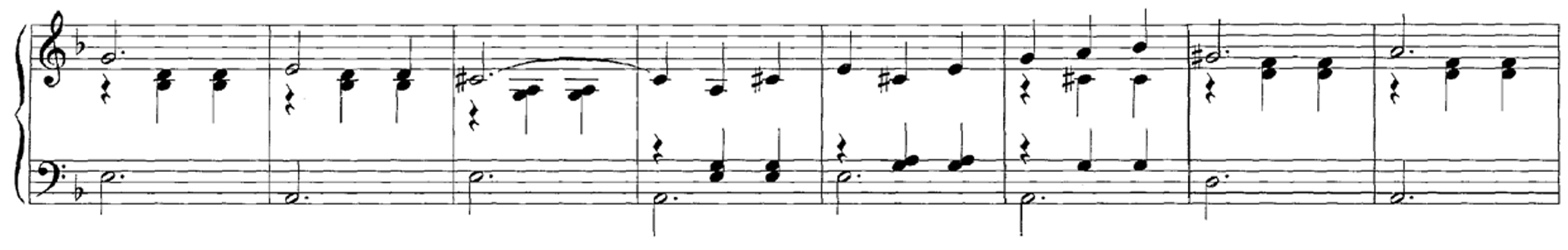Continuing the explanations from the FMP notebook on the Fourier tempogram, we show in the next figure a Fourier-based tempogram for the Shostakovich recording. Furthermore, we show optimal sinusoidal kernels plotted on top of the underlying novelty functions for various time-tempo pairs indicated as red points in the tempogram visualization.

In :
import os
import sys
import numpy as np
from numba import jit
import librosa
from scipy import signal
from scipy.interpolate import interp1d
from matplotlib import pyplot as plt
import matplotlib.gridspec as gridspec
import IPython.display as ipd

sys.path.append('..')
import libfmp.b
import libfmp.c2
import libfmp.c6

%matplotlib inline

fn_wav = os.path.join('..', 'data', 'C6', 'FMP_C6_F07_Shostakovich_Waltz-02-Section_IncreasingTempo.wav')
Fs = 22050

nov, Fs_nov = libfmp.c6.compute_novelty_spectrum(x, Fs=Fs, N=2048, H=512,
gamma=100, M=10, norm=True)
nov, Fs_nov = libfmp.c6.resample_signal(nov, Fs_in=Fs_nov, Fs_out=100)

N = 500 #corresponding to 5 seconds (Fs_nov = 100 Hz)
H = 10  #Hopsize leading to a tempogram resolution of 10 Hz
Theta = np.arange(30, 601) #Tempo range parameter
X, T_coef, F_coef_BPM = libfmp.c6.compute_tempogram_fourier(nov, Fs=Fs_nov, N=N, H=H,
Theta=Theta)
tempogram = np.abs(X)

t_nov = np.arange(nov.shape) / Fs_nov
coef_n = np.array([0, 10, 20, 30, 40, 50, 60])
coef_k = np.zeros(len(coef_n), dtype=int)

for i in range(len(coef_n)):
coef_k[i] = np.argmax(tempogram[:,coef_n[i]])

fig, ax, im = libfmp.b.plot_matrix(tempogram, T_coef=T_coef, F_coef=F_coef_BPM,
figsize=(6.5, 3), ylabel='Tempo (BPM)',
title='Fourier tempogram')
ax.plot(T_coef[coef_n], F_coef_BPM[coef_k], 'ro')

for i in range(len(coef_n)):
n = coef_n[i]
k = coef_k[i]
tempo = F_coef_BPM[k]
time = T_coef[n]
corr = np.abs(X[k,n])
kernel, t_kernel, t_kernel_sec = libfmp.c6.compute_sinusoid_optimal(X[k,n],
F_coef_BPM[k], n, Fs_nov, N, H)
title=r'Windowed sinusoid (t = %0.1f sec, $\tau$ = %0.0f BPM, corr = %0.2f)'% (time, tempo, corr)
libfmp.c6.plot_signal_kernel(nov, t_nov, 0.5*kernel, t_kernel_sec,
figsize=(6, 1.5), title=title)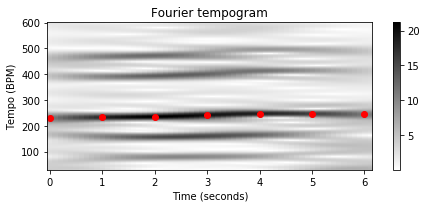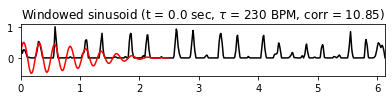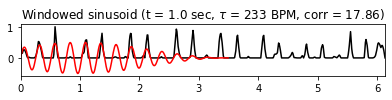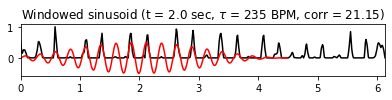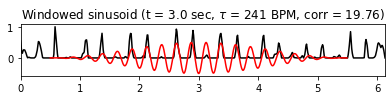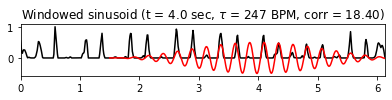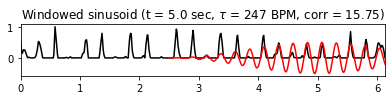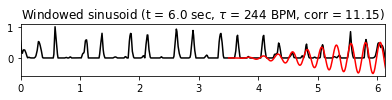## Definition of PLP Function¶

The estimation of optimal windowed sinusoids in regions with a strongly corrupt peak structure is problematic. This particularly holds in the case of small window sizes. To make the periodicity estimation more robust while keeping the temporal flexibility, the idea is to form a single function instead of looking at the sinusoids in a one-by-one fashion. To this end, we apply an overlap–add technique, where the optimal windowed sinusoids $\kappa_n$ are accumulated over all time positions $n\in\mathbb{Z}$. Furthermore, we only consider the positive part of the resulting function. More precisely, we define a function $\Gamma:\mathbb{Z}\to\mathbb{R}_{\geq 0}$ as follows:

\begin{equation} \Gamma(m) =\big|\textstyle \sum_{n\in \mathbb{Z}} \kappa_n(m)\big|_{\geq 0} \end{equation}

for $n\in\mathbb{Z}$, where we use the half-wave rectification. The resulting function is our mid-level representation referred to as PLP function (indicating the predominant local pulse). Continuing our Shostakovich example, we illustrate the steps of the PLP computation by showing the following plots:

• Novelty curve.
• Fourier tempogram with frame-wise maxima (shown for only seven time positions $n$ for visualization purposes).
• Optimal windowed sinusoids $\kappa_n$ using a window size of $5$ seconds (shown for only seven positions).
• Accumulation of optimal sinusoids over all time frames (overlap-add).
• Resulting PLP function $\Gamma$ obtained after half-wave rectification.
In :
L = nov.shape
N_left = N // 2
L_left = N_left
L_right = N_left
L_pad = L + L_left + L_right

t_nov = np.arange(nov.shape) / Fs_nov
coef_n = np.array([0, 10, 20, 30, 40, 50, 60])
coef_k = np.zeros(len(coef_n), dtype=int)

for i in range(len(coef_n)):
coef_k[i] = np.argmax(tempogram[:, coef_n[i]])

fig, ax = plt.subplots(5, 2, gridspec_kw={'width_ratios': [1, 0.05],
'height_ratios': [1, 2, 2, 1, 1]}, figsize=(8, 9))

libfmp.b.plot_signal(nov, Fs_nov, ax=ax[0, 0], color='k', title='Novelty function')
ax[0, 1].set_axis_off()

libfmp.b.plot_matrix(tempogram, T_coef=T_coef, F_coef=F_coef_BPM, ax=[ax[1,0], ax[1,1]],
title='Fourier tempogram', ylabel='Tempo (BPM)', colorbar=True)
ax[1, 0].plot(T_coef[coef_n], F_coef_BPM[coef_k], 'ro')

libfmp.b.plot_signal(nov, Fs_nov, ax=ax[2, 0], color='k', title='Novelty function with windowed sinusoids')
ax[2, 1].set_axis_off()

for i in range(len(coef_n)):
n = coef_n[i]
k = coef_k[i]
tempo = F_coef_BPM[k]
time = T_coef[n]
kernel, t_kernel, t_kernel_sec = libfmp.c6.compute_sinusoid_optimal(X[k, n], F_coef_BPM[k], n, Fs_nov, N, H)
nov_PLP[t_kernel] = nov_PLP[t_kernel] + kernel
ax[2, 0].plot(t_kernel_sec, 0.5 * kernel, 'r')
ax[2, 0].set_ylim([-0.6, 1])

libfmp.b.plot_signal(nov_PLP, Fs_nov, ax=ax[3, 0], color='r', title='Accumulated windowed sinusoids')
ax[3,1].set_axis_off()

nov_PLP[nov_PLP < 0] = 0
libfmp.b.plot_signal(nov_PLP, Fs_nov, ax=ax[4, 0], color='r', title='PLP function $\Gamma$')
ax[4, 1].set_axis_off()

plt.tight_layout()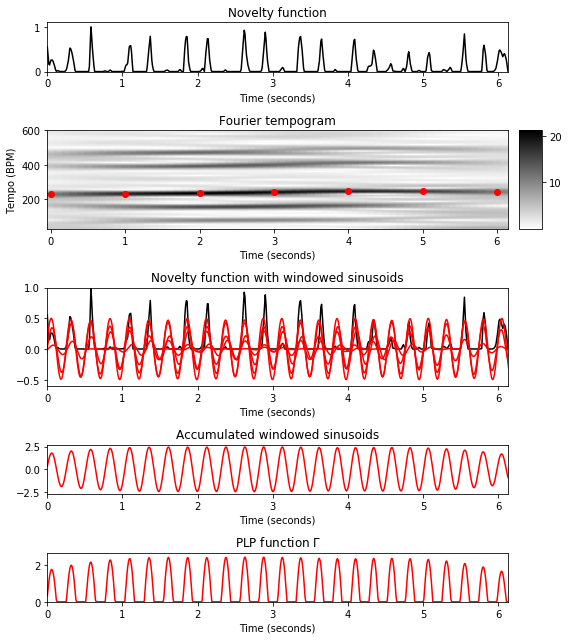Note how the maxima of the different windowed sinusoids align well not only with the peaks of the novelty function, but also with the maxima of neighboring sinusoids in the overlapping regions, which leads to constructive interferences. As indicated by the Fourier tempogram, the dominant tempo lies between $200$ and $250~\mathrm{BPM}$ throughout this excerpt with a slight tempo increase starting with roughly $\tau=225~\mathrm{BPM}$. The maximizing tempo values as well as the corresponding optimal windowed sinusoids are indicated for seven different time positions. Note that each of these windowed sinusoids tries to explain the locally periodic nature of the peak structure of the novelty function, where small deviations from the "ideal" periodicity and weak peaks are balanced out. Furthermore, note that the predominant pulse positions are clearly indicated by the peaks of $\Gamma$ even though some of these pulse positions are rather weak in the original novelty function. In this sense, the PLP function can be regarded as a local periodicity enhancement of the original novelty function, where the predominant pulse level is taken into account.

In the following code cell, we define a function that takes as input the complex-valued Fourier tempogram and outputs a PLP function, where the optimal windowed sinusoids $\kappa_n$ are accumulated over all tempogram frames $n$. The visualization shows the original novelty function as well as the resulting PLP function along with peaks obtained by some peak-picking strategy. Furthermore, a peak sonification via a click track added to the original audio recording generated.

In :
@jit(nopython=True)
def compute_plp(X, Fs, L, N, H, Theta):
"""Compute windowed sinusoid with optimal phase

Notebook: C6/C6S3_PredominantLocalPulse.ipynb

Args:
X (np.ndarray): Fourier-based (complex-valued) tempogram
Fs (scalar): Sampling rate
L (int): Length of novelty curve
N (int): Window length
H (int): Hop size
Theta (np.ndarray): Set of tempi (given in BPM)

Returns:
nov_PLP (np.ndarray): PLP function
"""
win = np.hanning(N)
N_left = N // 2
L_left = N_left
L_right = N_left
L_pad = L + L_left + L_right
M = X.shape
tempogram = np.abs(X)
for n in range(M):
k = np.argmax(tempogram[:, n])
tempo = Theta[k]
omega = (tempo / 60) / Fs
c = X[k, n]
phase = - np.angle(c) / (2 * np.pi)
t_0 = n * H
t_1 = t_0 + N
t_kernel = np.arange(t_0, t_1)
kernel = win * np.cos(2 * np.pi * (t_kernel * omega - phase))
nov_PLP[t_kernel] = nov_PLP[t_kernel] + kernel
nov_PLP[nov_PLP < 0] = 0
return nov_PLP

fn_wav = os.path.join('..', 'data', 'C6', 'FMP_C6_F07_Shostakovich_Waltz-02-Section_IncreasingTempo.wav')
Fs = 22050

nov, Fs_nov = libfmp.c6.compute_novelty_spectrum(x, Fs=Fs, N=2048, H=512,
gamma=100, M=10, norm=True)
nov, Fs_nov = libfmp.c6.resample_signal(nov, Fs_in=Fs_nov, Fs_out=100)

L = len(nov)
N = 500
H = 10
Theta = np.arange(30, 601)
X, T_coef, F_coef_BPM = libfmp.c6.compute_tempogram_fourier(nov, Fs=Fs_nov, N=N, H=H,
Theta=Theta)
nov_PLP = compute_plp(X, Fs_nov, L, N, H, Theta)

t_nov = np.arange(nov.shape) / Fs_nov
peaks, properties = signal.find_peaks(nov, prominence=0.02)
peaks_sec = t_nov[peaks]
libfmp.b.plot_signal(nov, Fs_nov, color='k', title='Novelty function with detected peaks');
plt.plot(peaks_sec, nov[peaks], 'ro')
plt.show()
x_peaks = librosa.clicks(peaks_sec, sr=Fs, click_freq=1000, length=len(x))
ipd.display(ipd.Audio(x + x_peaks, rate=Fs))

peaks, properties = signal.find_peaks(nov_PLP, prominence=0.02)
peaks_sec = t_nov[peaks]
libfmp.b.plot_signal(nov_PLP, Fs_nov, color='k', title='PLP function with detected peaks');
plt.plot(peaks_sec, nov_PLP[peaks], 'ro')
plt.show()
x_peaks = librosa.clicks(peaks_sec, sr=Fs, click_freq=1000, length=len(x))
ipd.display(ipd.Audio(x + x_peaks, rate=Fs))